# Team:Slovenia/ModelingDerivation

(Difference between revisions)
 Revision as of 22:10, 25 September 2012 (view source)Dusanv (Talk | contribs)← Older edit Revision as of 22:10, 25 September 2012 (view source)Dusanv (Talk | contribs) Newer edit → Line 455: Line 455:

- Deterministic model of the mutual repressor switch
+ Deterministic model of the mutual repressor switch
Deterministic model of the positive feedback loop switch Deterministic model of the positive feedback loop switch

# Deterministic model derivation - genetic switch

The deterministic model for the mutual repressor switch was derived in the following way.

We assumed the following binding site states were possible: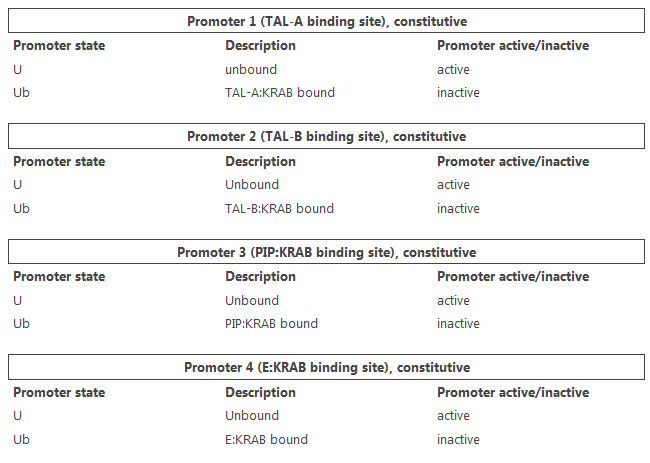Active promoter state is a state leading to gene expression.

Construct 1 promoter state transitioning is described by a reaction: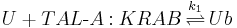Assuming equilibrium of binding and unbinding, we can write: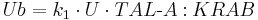The fractional occupancy of construct 1 promoter, f1, can be expressed as a ratio of active states to all states: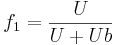The equations for other constructs take the same form.

It follows that the fractional occupancy for construct 1 promoter is: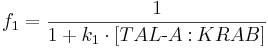To account for non-linearity, an exponent n1 was added, and the equation generalized to: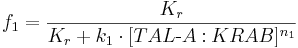Kr is the amount of TAL-A:KRAB it takes for f1 to be equal to 50%.

Derivation for constructs 2, 3 and 4 was similar except for different transcription factor names.

Fractional occupancies were then used to construct a set of ordinary differential equations representing each protein production. Because each protein can be produced from different constructs, production rates (including leaky rates) were summed together. E.g., because TAL-B:KRAB is produced from both constructs 1 and 3, fractional occupancies f1 and f3 were used and corresponding terms summed to obtain: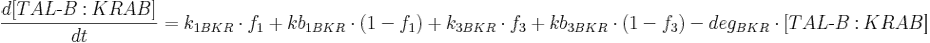Since construct 5 promoter has no binding sites and is active at all times, fractional occupancy of the promoter is equal to 1.

The positive feedback loop switch model was derived in a similar manner.

Deterministic model of the mutual repressor switch
Deterministic model of the positive feedback loop switch